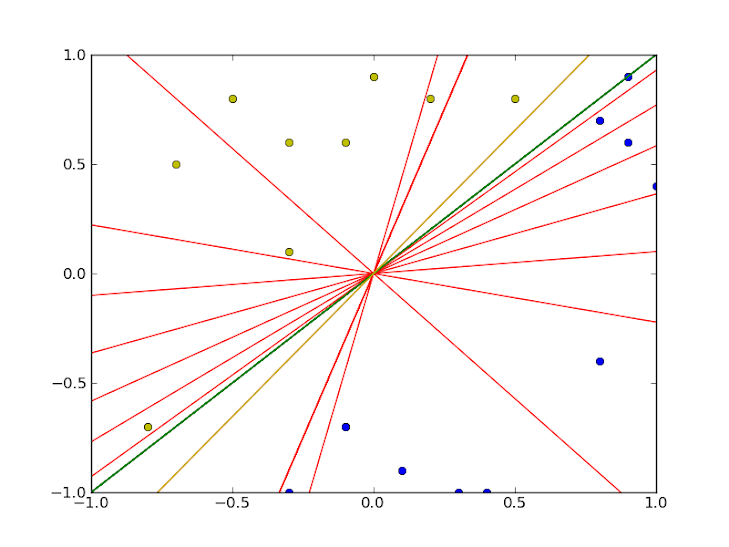LFD Book ForumDoubt on weights and pla

#1
 BarathMember Join Date: Jan 2013 Posts: 10Doubt on weights and pla

suppose i have a line f(x) = x , (for 2 dim) one side of which is +ve 1 and other side -ve 1..
Does this mean that target function is : [ -1 , 1] ? (since: -x + f(x) > 0 implies +ve 1 and -x + f(x) < 0 implies -ve 1)

and on running the PLA is the set of weights [-2 , 2] or any [-n, n] a valid solution for f(x) = x ? (since its just a constant to divide throughout?)

and when selecting random points, the PLA seems to run till infinity at times with
the same value of w repeating at regular intervals

am i getting the idea right here btw?
#2
 butterscotchCaltech Join Date: Jan 2013 Posts: 43Re: Doubt on weights and pla

If it is already known that the target function is linear and passes through (0,0) (such as f(x) = x), then it is known that bias term will be zero. [ -1 , 1] makes sense, which is indeed equivalnet to [-n,n]. However, if it's not the case, you do need the bias term (weight vector will have length of 3), otherwise the algorithm may not converge.
#3
 BarathMember Join Date: Jan 2013 Posts: 10Re: Doubt on weights and pla

Thanks for the idea about the bias it was really helpful! ... i tried a simple case for understanding better...

The PLA ran indefinitely because of the absence of bias (bias = 0 because of the target function i was having in my mind)
The problem was the random training points i generated based on the expected target function had a bit of a flaw that some points were present on the target function and not on either of its sides...further bias of 0 caused the PLA to generate lines that rotated about the origin alone..this at times prevented the algorithm to get to a solution and ran indefinitely!
#4
 BarathMember Join Date: Jan 2013 Posts: 10Re: Doubt on weights and pla

This is what happened without bias ..this time it terminated...
(many at times it did not as data points increased)
(Green = expected , yellow = final result , red = trials)Thread ToolsShow Printable VersionEmail this Page Display ModesLinear ModeSwitch to Hybrid ModeSwitch to Threaded ModePosting Rules You may not post new threads You may not post replies You may not post attachments You may not edit your posts BB code is On Smilies are On [IMG] code is On HTML code is Off Forum Rules
 Forum Jump User Control Panel Private Messages Subscriptions Who's Online Search Forums Forums Home General     General Discussion of Machine Learning     Free Additional Material         Dynamic e-Chapters         Dynamic e-Appendices Course Discussions     Online LFD course         General comments on the course         Homework 1         Homework 2         Homework 3         Homework 4         Homework 5         Homework 6         Homework 7         Homework 8         The Final         Create New Homework Problems Book Feedback - Learning From Data     General comments on the book     Chapter 1 - The Learning Problem     Chapter 2 - Training versus Testing     Chapter 3 - The Linear Model     Chapter 4 - Overfitting     Chapter 5 - Three Learning Principles     e-Chapter 6 - Similarity Based Methods     e-Chapter 7 - Neural Networks     e-Chapter 8 - Support Vector Machines     e-Chapter 9 - Learning Aides     Appendix and Notation     e-Appendices

All times are GMT -7. The time now is 06:49 AM.